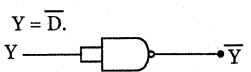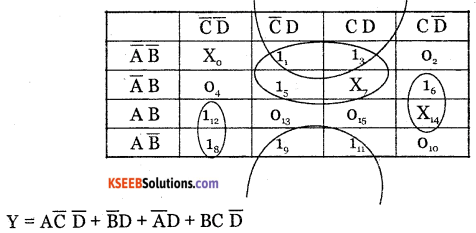# 2nd PUC Electronics Question Bank Chapter 10 Digital Electronics

Students can Download 2nd PUC Electronics Chapter 10 Digital Electronics Questions and Answers, Notes Pdf, 2nd PUC Electronics Question Bank with Answers helps you to revise the complete Karnataka State Board Syllabus and score more marks in your examinations.

## Karnataka 2nd PUC Electronics Question Bank Chapter 10 Digital Electronics

### 2nd PUC Electronics Digital Electronics One Mark Questions and Answers

Question 1.
What is an XOR gate?
The logic gate whose output is high only when odd number of inputs are high is known as an XOR gate

Question 2.
Draw the symbol of XOR gate?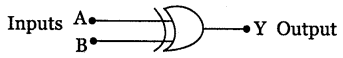Question 3.
Write the Boolean equation of XOR gate
$$\mathrm{Y}=\mathrm{A} \overline{\mathrm{B}}+\overline{\mathrm{A}} \mathrm{B}=\mathrm{A} \oplus \mathrm{B}$$Question 4.
Draw the symbol of XOR gate.

 Input Output A B Y= A ⊕ B 0 0 0 0 1 1 1 0 1 1 1 0

Question 5.
Draw the symbol of XNOR gate.Question 6.
Write the Boolean expression for the XNOR gate.
$$\mathrm{Y}=\mathrm{AB}+\overline{\mathrm{A}} \overline{\mathrm{B}} =\overline{\mathrm{A} \oplus \mathrm{B}}$$

Question 7.
Write the truth table of XNOR gate

 Inputs Output A B $$\mathrm{Y}=\overline{\mathrm{A} \oplus \mathrm{B}}$$ 0 0 1 0 1 0 1 0 0 1 1 1

Question 8.
Name the universal gates.
NAND gate and NOR gate.

Question 9.
How many two input NAND gates are required to produce two input OR function?
Three.

Question 10.
How many two input NOR gates must be used to produce two input OR function?
Two.

Question 11.
Why do we use digital codes?

Question 12.
What is BCD code?
BCD is a numeric code in which each digit of a decimal number is represented by a four bit binary number.Question 13.
What is a gray code?
Gray code is a non-weighted code which is used in analog to digital conversion.

Question 14.
Which are weighted codes?
8421 code, 2421 code.

Question 15.
Which are non-weighted codes?
Excess-3 code, Gray code.

Question 16.
Name the self complementing code.
Excess-3 code.

Question 17.
Convert 11012 into gray code.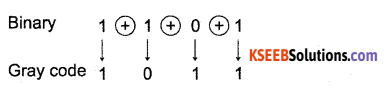Question 18.
Name the alpha numeric codes.
ASCII code, EBCDIC code.

Question 19.
Expand ASCII.
American Standard code for Information Interchange.

Question 20.
Expand EBCDIC.
Extended Binary Coded Decimal Interchange Code.

Question 21.
How many zone bits are there in EBCDIC?
4Question 22.
Half adder is a logic circuit which adds two bits and gives to outputs sum and carry.

Question 23.
Full adder is a combinational logic circuit that performs arithmetic sum of three input bits and gives as output sum and carry.

Question 24.
What is a half subtractor?
Half subtractor is a combinational logic circuit which performs the subtraction of two bits and gives difference and borrow.

Question 25.
Draw the block diagram of half adder.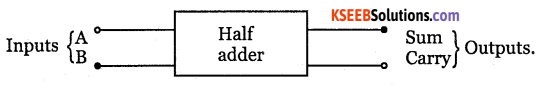Question 26.
Draw the block diagram of full adder.Question 27.
Draw the block diagram of half subtractorQuestion 28.
Write the Boolean expression for sum of half adder.
$$A \oplus B=A \bar{B}+\bar{A} B$$

Question 29.
Write the Boolean expression for sum of full adder.
A ⊕ B ⊕ Cin.Question 30.
Write the Boolean expression for the carry of half adder.
AB.

Question 31.
Write the Boolean expression for the carry of full adder.
Co = AB + BCin + Cin A.

Question 32.
Write the Boolean expression for difference of half subtractor.
A ⊕ B

Question 33.
Write the Boolean expression for borrow of half subtractor.
$$\overline{\mathrm{A}} \mathrm{B}$$

Question 34.
Define min term.
Min term is a special case product (AND) term containing all the input variables that make up a Boolean expression.

Question 35.
Define max term.
Max term is a special case sum (OR) term containing all the input variables that make up a Boolean expression.

Question 36.
Define SOP.
The Boolean expression containing all input variables in each of product term either in complemented or uncomplemented form is known as canonical sum of products expression.Question 37.
Define POS.
The Boolean expression containing all the input variables either in complemented or uncomplemented form in each of sum term is known as canonical POS expression.

Question 38.
What is canonical SOP equation?
It is an equation containing all input variables in each of product term either in complemented or uncomplemented form.

Question 39.
What is canonical POS equation?
It is an equation containing all input variables either in complemented or uncomplemented form in each of sum term.

Question 40.
What is a Karnaugh map?
Karnaugh map is a graphical method of simplification of Boolean expression.

Question 41.
What is meant by looping?
Looping is encircling of two adjacent 1 s on a K map.

Question 42.
What is a cell in K map?
A cell in a K map is a box which represents a particular combination of variables in its product form.

Question 43.
What is meant by redundant group?
A redundant group is a group in which all the 1 s in a group are over lapped by other groups.

Question 44.
What is a pair?
A group of adjacent 1 either horizontally or vertically but not diagonally on a K map is called a pair.

Question 45.
Quad is a group of four adjacent 1 s in a K-map.Question 46.
What is an octet?
An octet is a group of eight adjacent Is either in two rows or two columns in a K map.

Question 47.
How many variables does the pair eliminate?
One variable.

Question 48.
How many variables does the quad eliminate?
Two variables.

Question 49.
How many variables does the octet eliminate?
Three variables.

Question 50.
What is a don’t care condition?
An output condition that may be either 1 or 0 without affecting the operation of the system is called don’t care condition.

Question 51.
Name the universal gate used to realize AND-OR logic.
NAND gate.Question 52.
Name the universal gate used to realize OR-AND logic.
NOR gate

Question 53.
What is a sequential logic circuit?
Sequential logic circuits are those circuits whose output level depends on present levels of input and previous input levels.

Question 54.
What is a flip flop?
A flip flop is a bistable multivibrator which has two stable states. It is a basic memory element which can store one bit information.

Question 55.
Is the flip flop a bistable device?
Yes Flip flop is a bistable device.

Question 56.
Define a clock pulse.
A clock is a periodic train of pulses or square waves which acts as a control signal. The output changes state only when clock makes transition.

Question 57.
Draw the logic circuit of an unclocked SR flip flop.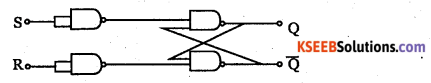Question 58
Draw the logic diagram of a D flip flop.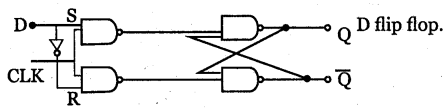Question 59.
Draw the logic diagram of a JK flip flop.Question 60.
Draw the logic diagram of T nip flop.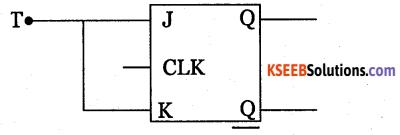Question 61.
What is a register?
A register is a set of flip flops used to store binary data.

Question 62.
Which is the line used to transfer data in and out of a PISO shift register?

Question 63.
What is a counter?

### 2nd PUC Electronics Digital Electronics Two Marks Questions and Answers

Question 1.
What is an XOR gate? Write its truth table.
XOR gate is a logic gate whose output is HIGH only when odd number of inputs are HIGH.

 Input Output A B Y = A + B 0 0 1 0 1 0 1 0 0 1 1 1

Question 2.
What is an XNOR gate? Write its truth table.
XNOR gate is a logic circuit whose output is HIGH only the inputs are same

 Input Output A B Y = A + B 0 0 1 0 1 0 1 0 0 1 1 1Question 3.
Draw the pin diagram of IC 7400.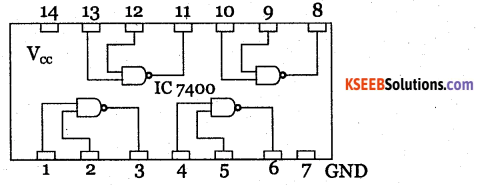Question 4.
Draw the pin diagram of IC 7402.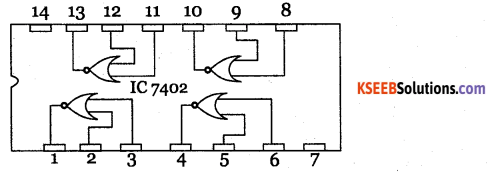Question 5.
Explain the term universal gates.
NAND gate and NOR gate are called universal gates because all the logic gates can be realised using these gates.

Question 6.
Realise XNOR gate using only NOR gates.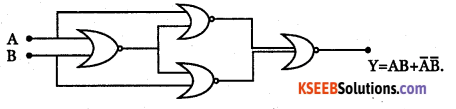Question 7.
What is the most important characteristic of gray code? Explain.
Gray code changes only by one bit, each time the decimal number is incremented.

 Decimal digit BCD Gray 0 0000 0000 1 0001 0001 2 0010 0011 3 0011 0010 4 0100 0110 5 0101 0111

Question 8.
Distinguish between excess-3 code and BCD code
Excess-3 code is a non-weighted self complementing code. It is obtained by adding 3 to each digit in decimal number. BCD code is a weighted code. Each decimal number is represented by a separate group of four bits.Question 9.
Convert gray code 1000 into binary using XOR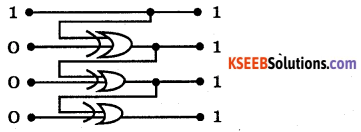Question 10.
Full adder is combinational circuit which adds three bits and gives sum and carry. Half adder adds two bits and gives sum and carry.

Question 11.
Write the truth table and draw the timing diagram of a half adder.

 Inputs Outputs A B Sum Y = A⊕B Carry Y = AB 0 0 0 0 0 1 1 0 1 0 1 0 1 1 0 1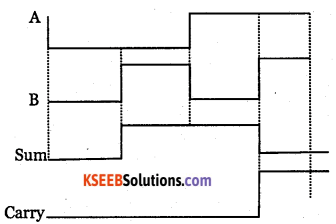Question 12.
Write the truth table and draw the timing diagram of a full adder.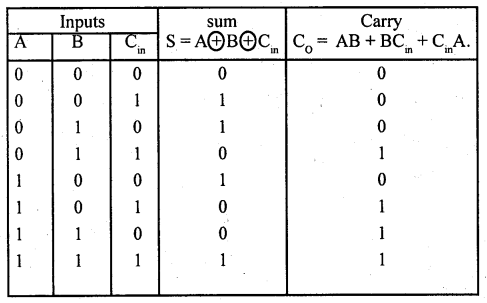Question 13.
Realise the full adder using two half adders and a OR gate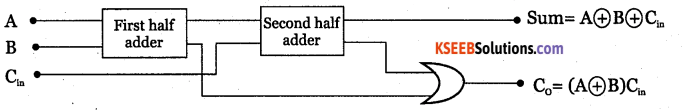Question 14.
Distinguish between SOP and POS equations.
SOP equation is equation containing all the input variables in each of product term either in complemented or uncomplemented form.

POS equation is the equation containing all the input variables either in complemented or uncomplemented form in each of sum term.

Question 15.
Convert $${ AB }+\overline { { C } }$$ into canonical SOP.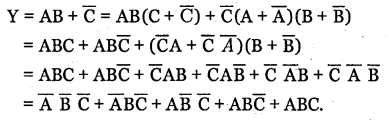Question 16.
Convert $$(\mathbf{A}+\mathbf{C})(\mathbf{B}+\overline{\mathbf{C}})$$ into canonical POSQuestion 17.
Distinguish between min term and max term.
A max term is a special case sum term containing all input variables that make up a Boolean expression. A min term is a special case product term containing all input variable that make up a Boolean expression.

Question 18.
Write the truth tables for 3 variable input with min term designations.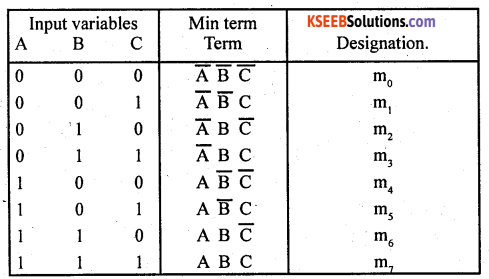Question 19.
Explain the necessity of elimination redundant groups in a K-map.
A redundant group is a group in which all the 1 s in a group are overlapped by other groups. Redundent group should be eliminated before writing simplified Boolean equation using k map. The elimination of redundant groups results in a simple logic circuit.

Question 20.
Explain don’t care condition.
An output condition that may be treated as either 1 or 0 without affecting the operation is called don’t care condition.

When simplifying a logic expression using a K-map, a don’t care condition can be treated as a 1 if it contributes to the simplification and can be treated as 0 if it does not contribute to the simplification.

Question 21.
Distinguish between AND-OR logic and OR-AND logic.

 AND OR logic OR-AND logic 1. It is used in simlifying SOP expression. 1. It is used in simplifying POS expression. 2. It is realised only using NAND gates. 2. It is realised only using NOR gates.

Question 22.
Draw the 4 variable K-map with min term designationsQuestion 23.
Write the truth table for the expression $$\mathbf{Y}=\mathbf{A} \overline{\mathbf{B}}+\overline{\mathbf{A}} \mathbf{B}$$Question 24.
Draw the AND-OR logic circuit for the expression $$\mathbf{Y}=\overline{\mathbf{A}} \mathbf{B}+\overline{\mathbf{B}}$$Question 25.
Draw the logic circuit for the expression  $$\mathbf{Y}=\mathbf{A} \mathbf{C}+\overline{\mathbf{C}}$$ using only basic gates.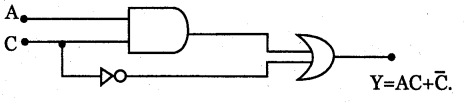Question 26.
Draw the logic circuit for the expression $$\mathbf{Y}=\mathbf{A} \mathbf{B}+\overline{\mathbf{B}}$$ using only NAND gates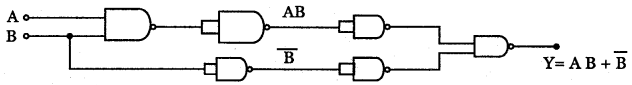Question 27.
Distinguish between combinational and sequential logic circuits.
Combinational logic circuits are circuits whose output level at any instant of time is dependent only on present levels of inputs at that time. These circuits do not have memory.

Sequential logic circuits are those circuits whose output levels at any instant of time depend not only on the present input state but also on previous input states. These circuits have memory.

Question 28.
Distinguish between SR and JK flip flop.

 SR Flip Flop JK Flip Flop 1. When S = R = 1, it is in forbidden state. 1.When J=-K= 1, it toggles. 2. It can not be used for any application. 2. It can be used in most of applications.

Question 29.
What is race around condition? How is it etiminated?
Toggling of the output more than once during the same clock pulse is called race around condition. It can be eliminated using an RC network (edge triggering) at the clock input or by using Master-slave JK flip flop.

Question 30.
Mention the types of shift registers.

• Serial-in, serial-out.
• Serial-in, parallel-out
• Parallel-in, serial-out.
• Parallel-in, parallel-out.

Question 31.
Draw the logic diagram of SISO shift register.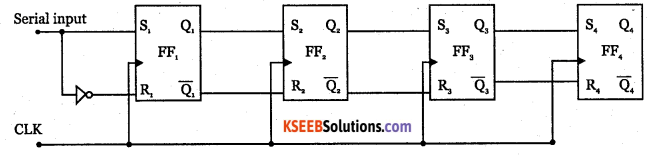Question 32.
Draw the logic diagram of SIPO shift registor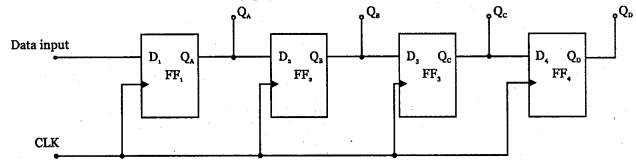Question 33.
Draw the logic diagram of PISO shift register.Question 34.
Draw the logic diagram PIPO shift register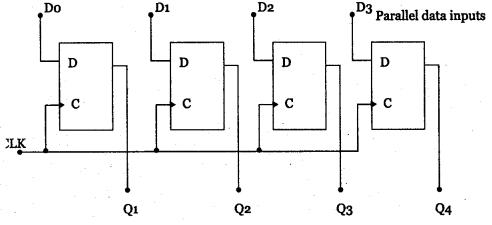Question 35.
Mention a broad classification of counters.
Counters are classified as synchronous counters and asynchronous counters.

### 2nd PUC Electronics Digital Electronics Three Marks Questions and Answers

Question 1.
What is an XOR gate? Realise logic expression Y=A⊕B⊕C using 2 input two XOR gates.
XOR gate is a logic circuit whose output is HIGH only when odd number of inputs are HIGH.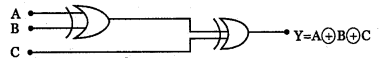Question 2.
Construct an XOR gate Using only NAND gates and write the Boolean expression at the output of each gate.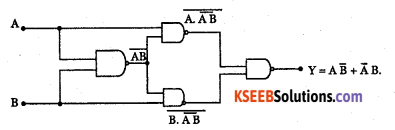Question 3.
Write the table of 2-4-2-1 code and show that it is a self complementing code

 Decimal Digit 2-4-2-1 Code 0 0000 1 0001 2 0010 3 0011 4 0100 5 0101 6 0.100 7 1101 8 1110 9 1111

1’s complement of 1100(610) = 0011 = 310
= 9’s complement of 6
∴ 2421 code is self complementing codeQuestion 4.
Write ASCII codes for decimal numbers 0 and 9

 Decimal Number ASCIlCode 0 48 1 49 2 50 3 51 4 52 5 53 6 54 7 55 8 56 9 57

Question 5.
Draw logic symbol, timing diagram and truth table of
(ii) Half subtractor.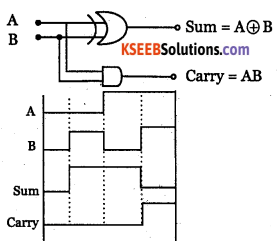Inputs Sum Carry A B Y = A +B Y = AB 0 0 0 0 0 1 1 0 1 0 1 0 1 1 0 1

(ii) Half subtractor.Inputs Outputs A B Difference Borrow 0 0 0 0 0 1 1 1 1 0 1 0 1 1 0 0

Question 6.
Realise the half adder using only NAND gates and write the Boolean expression at the output of each gate.Question 7.
Write the truth table and draw the timing diagram of full adder.

 Inputs Outputs A B Sum Y = A + B Carry Y = AB 0 0 0 0 0 1 1 0 1 0 1 0 1 1 0 1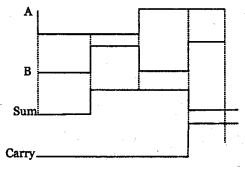Question 8.
Realise full adder using two XOR, two AND gates and an OR gate.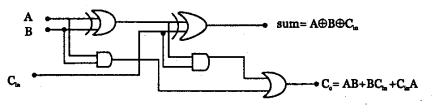Question 9.
Write A+BC+AB into its canonical SOP and write the expression in min term designation.Question 10.
Simplify the Boolean expression Y=∑m(0,2,4,6,8,10,ll,12,14,15) +∑d(9,13) using k-map.Question 11.
List the different types of flip flops.

• SR flip flop
• D flip flop
• JKflip flop
• Master-Slave JK flip flop.

Question 12.
Draw the logic symbol, truth table and timing diagram of D flip flop.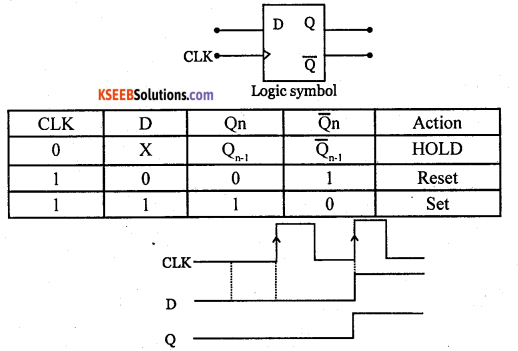Question 13.
Draw the logic symbol, truth table and timing diagram of T flip flop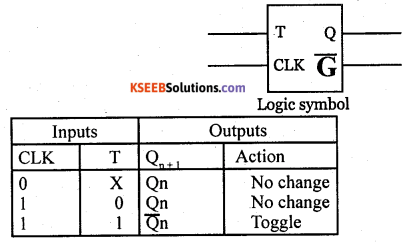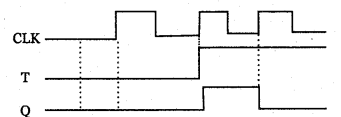Question 14.
Mention few applications of flip flops.

• Transfer and storage of data.
• Frequency division.
• Counters
• Detection of input sequence of data.

Question 15.
Write truth table, timing diagram and logic diagram of SISO register.Question 16.
Draw the logic diagram and counting sequence of a four bit synchronous up counter.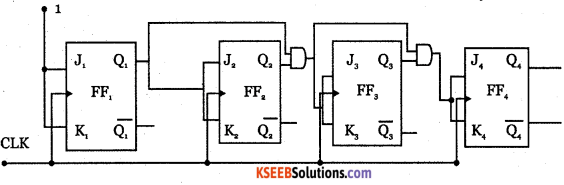### 2nd PUC Electronics Digital Electronics Five Marks Questions and Answers

Question 1.
Explain the universal property of NAND gate and realize AND, OR, NOT and XOR gates with their respective truth tables.
NAND is called universal gate because other logic gates can be realised using NAND gates.
(1) AND gate from NAND gates: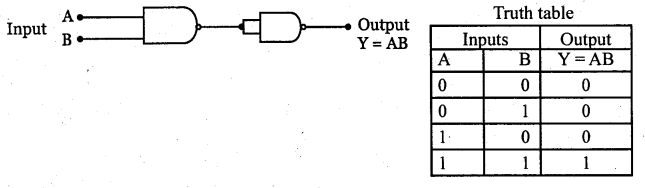Inputs Output A B Y = AB 0 0 0 0 1 0 1 0 0 1 1 1

(2) OR gate from NAND gates: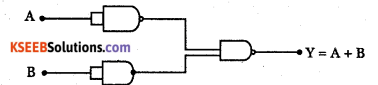Inputs Output A B Y =A+B 0 0 0 0 1 1 1 0 1 1 1 1

(3) NOT gate from NAND gate: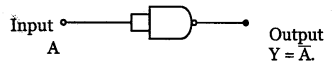Inputs Output A $$\overline{\mathbf{A}}$$ 0 1 1 0

(4) XOR gate from NAND gates: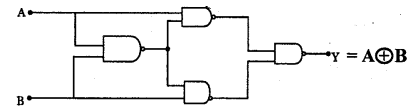Inputs Output A B Y 0 0 0 0 1 1 1 0 1 1 1 0Question 2.
What is a half subtractor? Explain its working with a truth table, logic diagram and timing diagram.
Half subtractor is a logic circuit which subtracts two bits and gives difference and borrow.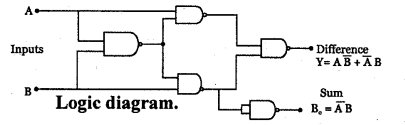Inputs Output A B Difference Borrow 0 0 0 0 0 1 1 1 1 0 1 0 1 1 0 0

Question 3.
What is full adder? Explain its working with respect to three input XOR gate and basic gates with the help of truth table and Boolean expression.
Full adder is a combinational logic circuit that performs arithmetic sum of three bits and gives their sum and carry.Question 4.
Simplify the Boolean expression Y=Em(0,4,5,7) using k-map. Realise simplified expression by using both AND-OR logic and NAND-NAND gates.Question 5.
Convert $$Y\quad =\bar { AB } +BC+\bar { BD }$$ into its canonical SOP form and write the truth table for the corresponding min term designation.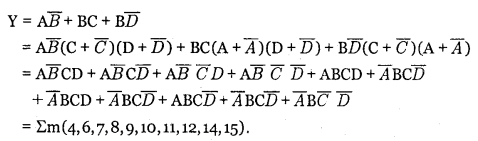Question 6.
With a logic circuit, explain working of un-clocked SR flip flop built using NAND gates. Draw its timing diagram and truth table.It is constructed using inverters inserted into inputs of cross coupled NAND gates.
Working:

1. When S=0, R=0, it does not respond and hence outputs Q and Q will remain in their previous state. This is called HOLD condition.
2. When S=1 and R=0, the output Q and Q change to 1 and 0. This condition is called SET state.
3. When S=0, R=1, the output Q and Q change to 0 and 1. This condition is called RESET state.
4. When S=1 and R=l, it drives output Q and Q both to HIGH which is FORBIDDEN or INVALID condition.Question 7.
Explain the working of clocked Jk flip flop with its logic diagram ,truth table and timing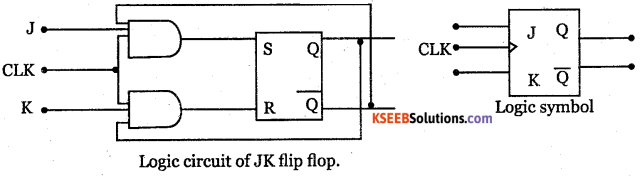1. When J = 0, K= 0, the S and R inputs are both at 0 and hence the output is in the HOLD state.
2. When J = 0, K = 1, S input is at 0 while R can be either at 0 or 1, but output is always in the RESET state.
3. When J = 1, K = 0, S input can be 0 or 1 but R input is always at logic 0 and hence the output remains at a stable SET state.
4. When J = 1, K = 1 the Flip flop goes to complementary’ state of previous output i.e, flip flop togglesQuestion 8.
Explain the working of SISO shift register with relevant diagrams.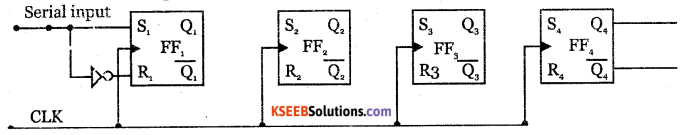With four SR flip flops it can store upto 4 bits of data. Serial data is applied to S input of the first flip flop.

When serial data is transferred into the register, each new bit is clocked into first flip flop at the positive edge of each clock pulse. The bit that was stored by first flip flop is transferred to second flip flop and so on.

The shifting out of stored data 0101 serially from the register requires four clock pulses, which is represented as in the following truth table.

Question 9.
Explain the working of a four bit synchronous up counter using relevant diagrams.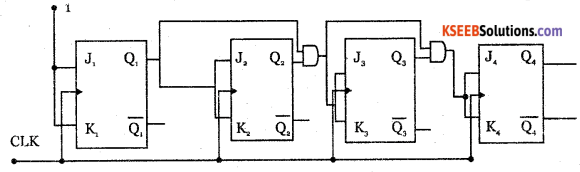Counter is a logic circuit used for counting the pulses. It is a set of flip flops whose state changes in response to pulses applied to their inputs.

Counters are of two types:

• Synchronous counters and
• Asynchronous counters.In a synchronous circuit, the clock signal is applied to all flip flops simultaneously.

Question 10.
Simplify the Boolean equation Y=∑m(0,2,4,8,10) +∑d(12,14) using K-map. Draw the NAND gate equivalent circuit to realise simplified equation.
∑m(0,2,4,8,10) +∑d(12,14)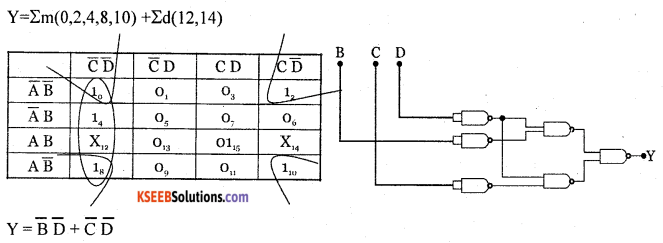Question 11.
Simplify the Boolean equation Y= ∑m(4,5,7,9,11,12,13,15) +∑d(1,3,8) using k-map. Draw the NAND gate equialent circuit to realise the simplified equation.
Y=∑d_(4,5,7,9,11,12,13,15) +∑d (1,3,8)
$$Y=B \bar{C}+D$$Question 12.
Simplify Boolean equation Y=∑d (0,2,6,8,10,12,14) +∑d (4,9,13) using K map. Draw the NAND gate equivalent circuit to realise simplified equation.